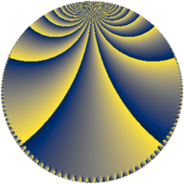# Properties

 Label 384.5.hLevel $384$ Weight $5$ Character orbit 384.h Rep. character $\chi_{384}(65,\cdot)$ Character field $\Q$ Dimension $64$ Newform subspaces $8$ Sturm bound $320$ Trace bound $3$

# Related objects

## Defining parameters

 Level: $$N$$ $$=$$ $$384 = 2^{7} \cdot 3$$ Weight: $$k$$ $$=$$ $$5$$ Character orbit: $$[\chi]$$ $$=$$ 384.h (of order $$2$$ and degree $$1$$) Character conductor: $$\operatorname{cond}(\chi)$$ $$=$$ $$24$$ Character field: $$\Q$$ Newform subspaces: $$8$$ Sturm bound: $$320$$ Trace bound: $$3$$ Distinguishing $$T_p$$: $$5$$, $$11$$

## Dimensions

The following table gives the dimensions of various subspaces of $$M_{5}(384, [\chi])$$.

Total New Old
Modular forms 272 64 208
Cusp forms 240 64 176
Eisenstein series 32 0 32

## Trace form

 $$64 q + O(q^{10})$$ $$64 q + 8000 q^{25} - 1984 q^{33} + 27968 q^{49} - 2240 q^{57} + 14720 q^{73} + 17728 q^{81} + 11264 q^{97} + O(q^{100})$$

## Decomposition of $$S_{5}^{\mathrm{new}}(384, [\chi])$$ into newform subspaces

Label Dim $A$ Field CM Traces $q$-expansion
$a_{2}$ $a_{3}$ $a_{5}$ $a_{7}$
384.5.h.a $2$ $39.694$ $$\Q(\sqrt{6})$$ $$\Q(\sqrt{-6})$$ $$0$$ $$-18$$ $$0$$ $$0$$ $$q-9q^{3}+\beta q^{5}-5\beta q^{7}+3^{4}q^{9}-142q^{11}+\cdots$$
384.5.h.b $2$ $39.694$ $$\Q(\sqrt{-2})$$ $$\Q(\sqrt{-2})$$ $$0$$ $$-14$$ $$0$$ $$0$$ $$q+(-7-\beta )q^{3}+(17+14\beta )q^{9}+46q^{11}+\cdots$$
384.5.h.c $2$ $39.694$ $$\Q(\sqrt{-2})$$ $$\Q(\sqrt{-2})$$ $$0$$ $$14$$ $$0$$ $$0$$ $$q+(7+\beta )q^{3}+(17+14\beta )q^{9}-46q^{11}+\cdots$$
384.5.h.d $2$ $39.694$ $$\Q(\sqrt{6})$$ $$\Q(\sqrt{-6})$$ $$0$$ $$18$$ $$0$$ $$0$$ $$q+9q^{3}+\beta q^{5}+5\beta q^{7}+3^{4}q^{9}+142q^{11}+\cdots$$
384.5.h.e $4$ $39.694$ $$\Q(\sqrt{-2}, \sqrt{-5})$$ None $$0$$ $$-12$$ $$0$$ $$0$$ $$q+(-3-\beta _{1})q^{3}+\beta _{2}q^{5}+\beta _{2}q^{7}+(-63+\cdots)q^{9}+\cdots$$
384.5.h.f $4$ $39.694$ $$\Q(\sqrt{-2}, \sqrt{-5})$$ None $$0$$ $$12$$ $$0$$ $$0$$ $$q+(3-\beta _{1})q^{3}-\beta _{2}q^{5}+\beta _{2}q^{7}+(-63+\cdots)q^{9}+\cdots$$
384.5.h.g $16$ $39.694$ $$\mathbb{Q}[x]/(x^{16} + \cdots)$$ None $$0$$ $$0$$ $$0$$ $$0$$ $$q-\beta _{4}q^{3}+\beta _{8}q^{5}+\beta _{7}q^{7}+(-7-\beta _{5}+\cdots)q^{9}+\cdots$$
384.5.h.h $32$ $39.694$ None $$0$$ $$0$$ $$0$$ $$0$$

## Decomposition of $$S_{5}^{\mathrm{old}}(384, [\chi])$$ into lower level spaces

$$S_{5}^{\mathrm{old}}(384, [\chi]) \cong$$ $$S_{5}^{\mathrm{new}}(24, [\chi])$$$$^{\oplus 5}$$$$\oplus$$$$S_{5}^{\mathrm{new}}(96, [\chi])$$$$^{\oplus 3}$$$$\oplus$$$$S_{5}^{\mathrm{new}}(192, [\chi])$$$$^{\oplus 2}$$